# 无监督学习中的无监督特征学习、聚类和密度估计

## 无监督学习概述

• 无监督特征学习（Unsupervised Feature Learning）

• 密度估计（Density Estimation）

• 聚类（Clustering）

## 无监督特征学习

• 主成分分析
• 稀疏编码
• 自编码器

### 主成分分析

#### PCA中的最大可分性思想

PCA降维，用原始样本数据中最主要的方面代替原始数据，最简单的情况是从2维降到1维，如下图所示，我们希望找到某一个维度方向，可以代表两个维度的数据，图中列了两个方向 $u_1, u_2$，那么哪个方向可以更好的代表原始数据呢？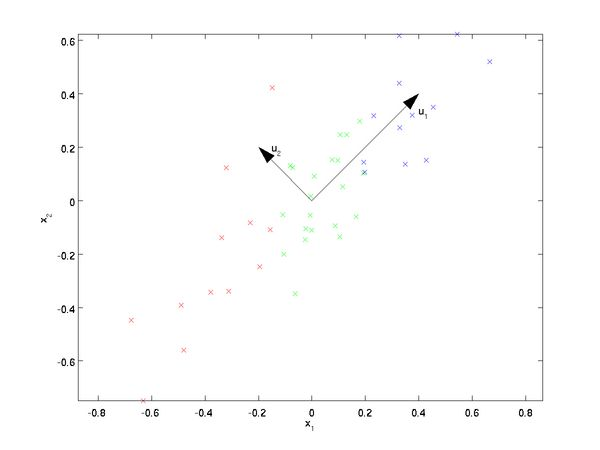#### 基变换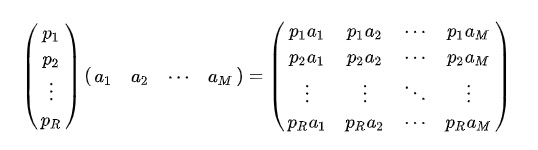#### 方差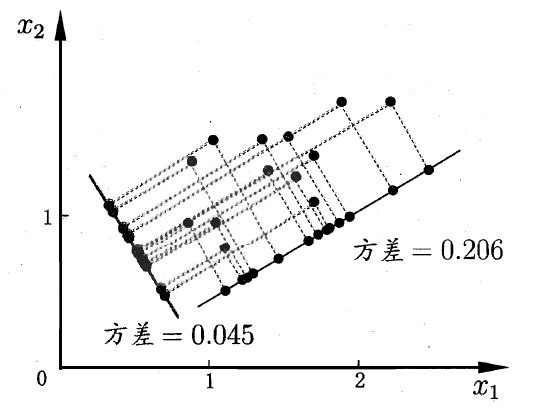$Var(a) = \frac{1}{m} \sum_{i=1}^{m} (a_i - \mu)^2$

$Var(a) = \frac{1}{m} \sum_{i=1}^{m} (a_i)^2$

• 对原始数据进行（线性变换）基变换可以对原始样本给出不同的表示
• 基的维度小于样本的维度可以起到降维的作用，
• 对基变换后新的样本求其方差，选取使其方差最大的基

#### 协方差

$Cov(a,b)= \frac{1}{m} \sum_{i=1}^{m}a_i b_i$

#### 协方差矩阵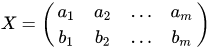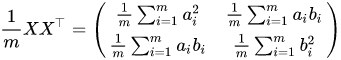#### 协方差矩阵对角化

$D=\frac{1}{m}YY^T \\ = \frac{1}{m}(PX)(PX)^T \\ = \frac{1}{m} PXX^TP^T \\ =P(\frac{1}{m} XX^T)P^T \\ = PCP^T \\ =P \begin{pmatrix} \frac{1}{m} \sum_{i=1}^{m} a_i^2 & \frac{1}{m} \sum_{i=1}^{m} a_i b_i \\ \frac{1}{m} \sum_{i=1}^{m} a_ib_i & \frac{1}{m} \sum_{i=1}^{m} b_i^2 \end{pmatrix} P^T$

$\underset{P}{max} \, tr(PCP^T) \\ s.t. \,PP^T=I$

$J(P) = tr(PCP^T) + \lambda(PP^T - I)$

$CP^T = (- \lambda) P^T$

#### PCA算法流程

• 输入：n维的样本集 $X=(x_i, x_2,...,x_m)$，要降维到的维数$n'$
• 输出：降维后的维度Y
1. 对所有的样本集去中心化 $x_i = x_i - \frac{1}{m} \sum_{j=1}^{m}x_j$
2. 计算样本的协方差矩阵$C = \frac{1}{m}XX^T$
3. 求出协方差矩阵对应的特征值和对应的特征向量
4. 将特征向量按照特征值从大到小，从上到下按行排列成矩阵，取前k行组成矩阵P
5. $Y=PX$即为降维到K维之后的数据

#### PCA算法总结

PCA算法的主要优点：

• 仅仅需要以方差衡量信息量，不受数据集意外因素的影响
• 各主成分之间正交，可消除原始数据各成分间的相互影响的因素
• 方法设计简单，主要运算是特征值分解，易于实现

PCA算法的主要缺点：

• 主成分各个特征维度的含义具有一定的模糊性，不如原始样本特征的可解释性强
• 方差小的非主成分也可能包含对样本差异的重要信息，因降维丢弃可能会对后续数据处理有影响
• 当样本特征维度较大时，需要巨大的计算量（比如，10000*10000，这时候就需要SVD[奇异值分解]，SVD不仅可以得到PCA降维的结果，而且可以大大的减小计算量）

### 稀疏编码

#### 稀疏编码（Sparse Coding）介绍

$x = \sum _{i=1}^{p} z_i a_i = Az$

“过完备”基向量一般指的是基向量个数远大于其支撑空间维度，因此这些基向量一般是不具备独立，正交等性质。

$L(A,Z)= \sum _{n=1}^{N}( || x^n - Az^n || ^2 + \eta \rho (z^n))$

$\rho(z) = \sum _{i=1}^{p} I(|z_i| > 0)$
$l_0$范数不满足连续可导，因此很难进行优化，在实际中，稀疏性衡量函数通常选用$l_1$范数
$\rho(z) = \sum _{i=1}^{p} |z_i|$

$\rho(z) = \sum _{i=1}^{p} log(1+z_i^2)$

$\rho(z) = \sum _{i=1}^{p} -exp(-z_i^2)$

#### 训练方法

（1）固定基向量A，对每个输入$x^n$ ，计算其对应的最优编码（原内容为减去稀疏性衡量函数，觉得不对）
$\underset{x^n}{min} || x^n - Az^n ||^2 + \eta \rho (z^n), \forall n \in [1,N]$
（2）固定上一步得到的编码$z^1, ...,z^N$，计算其最优的基向量
$\underset{A}{min} \sum _{i=1}^{N} ( || x^n - Az^n ||^2 ) + \lambda \frac{1}{2} ||A||^2$

### 自编码器

$L = \sum_{n=1}^{N} || x^n -g(f(x^n)) ||^2 = \sum || x^n -f \cdot g(x^n) ||^2$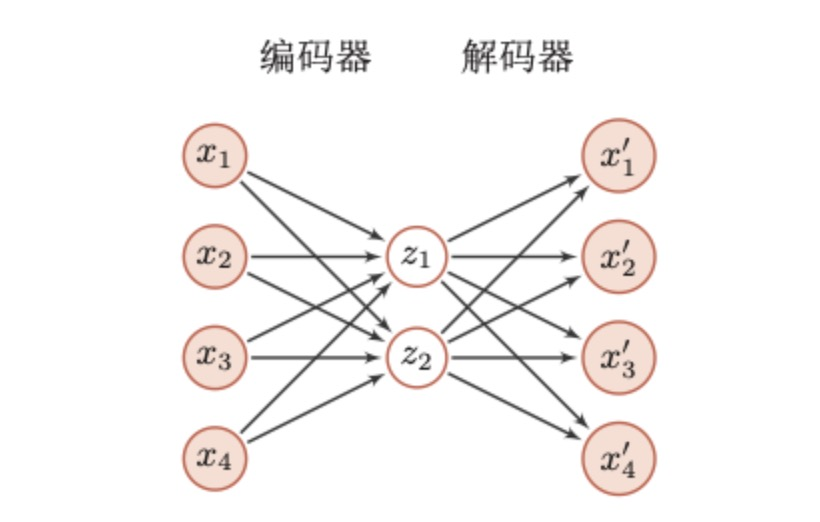$z = s(W^1 x + b^l)$

$x' = s(W^2 z + b^l)$

$L = \sum_{n=1}^{N} || x^n -x^{'n} ||^2 + \lambda ||W||_F^2$

### 降噪自编码器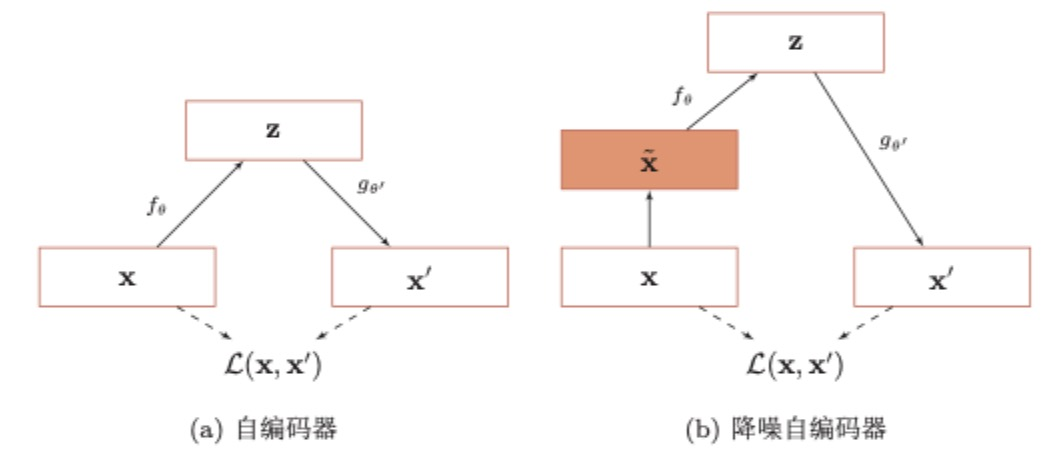## 概率密度估计

• 参数密度估计
• 非参数密度估计

### 参数密度估计

$D = {\{x^n\}}_{i=1}^{N}$为某个未知分布中独立抽取的N个训练样本，假设这些样本服从一个概率分布函数$p(x|\theta)$，其对数似然函数为：
$log\,p(D|\theta) = \sum_{n=1}^{N}log\,p(x^n|\theta)$

$\theta ^{ML} = \underset{\theta}{arg\,max } \sum_{n=1}^{N}log\,p(x^n|\theta)$

#### 正态分布中的参数密度估计

$X \sim N(\mu,\sigma^2) = \frac{1}{ \sqrt{2\pi} \sigma^2} e^{- \frac{(x-\mu)^2}{2\sigma^2}}$
$\mu,\sigma^2$的最大似然估计量。

$X$的概率密度为：
$f(x;\mu,\sigma^2) = \frac{1}{ \sqrt{2\pi} \sigma^2} e^{- \frac{(x-\mu)^2}{2\sigma^2}}$

$L(\mu,\sigma^2) = \prod_{i=1}^{N} \frac{1}{ \sqrt{2\pi} \sigma^2} e^{- \frac{(x-\mu)^2}{2\sigma^2}} \\ = (2\pi)^{-\frac{N}{2}} (\sigma^2)^{-\frac{N}{2}} e^{(-\frac{1}{2\sigma^2} \sum_{i=1}^{N} (x_i - \mu)^2)}$

$Ln\, L =-\frac{N}{2} ln(2\pi)-\frac{N}{2} ln(\sigma^2) - \frac{1}{2\sigma^2} \sum_{i=1}^{N}(x_i - \mu)^2$

$\left\{\begin{matrix} \frac{\partial }{\partial \mu }ln\, L = \frac{1}{\sigma^2} (\sum_{i=1}^{N} x_i -N\mu ) =0 & \\ \\ \frac{\partial }{\partial \sigma^2 }ln\, L = - \frac{N}{2\sigma^2} + \frac{1}{ (2\sigma^2)^2} \sum_{i=1}^{N}(x_i-\mu)^2 =0& \end{matrix}\right.$

$\tilde{\mu} = \bar{X},\tilde{\sigma^2}=\frac{1}{N}(x_i - \bar{x})^2$

#### 多项分布中的参数密度估计

$p(x|\mu) = \prod_{k=1}^{K}\mu_k ^{x_K}$

$log(D|\mu) = \sum_{n=1}^{N} \sum_{k=1}^{K} x_n ^k log (\mu _k)$

$\underset{\mu, \lambda}{ max} \sum_{n=1}^{N} \sum_{k=1}^{K} x_k ^n log(\mu_k) + \lambda (\sum_{k=1}^{K} \mu_k -1)$

$\mu_k ^{ML} = \frac{m_k}{N}, 1 \leq N \leq K$

• （1）模型选择问题，即如何选择数据分布的密度函数，实际的数据分布往往是非常复杂的，而不是简单的正态分布或者多项分布。
• （2）不可观测变量问题，即我们用来训练数据的样本只包含部分的可观测变量，还有一些非常关键的变量是无法观测的，这导致我们很难估计数据的真实分布。
• （3）维度灾难问题，即高维的参数估计十分困难。随着维度的增加，估计参数所需要的样本量呈指数增加。在样本不足时会出现过拟合。

#### 非参数密度估计

$P_K = \binom{N}{K}P^K(1-P)^{1-K}$

• （1）固定区域大小V，统计落入不同区域的数量，这种方式包括直方图和核方法两种
• （2）改变区域大小，以使得落入每个区域的样本数量为K，这种方法成为K近邻方法
##### 直方图方法

$p_m = \frac {K_m}{N\Delta m},1 \leq m \leq M$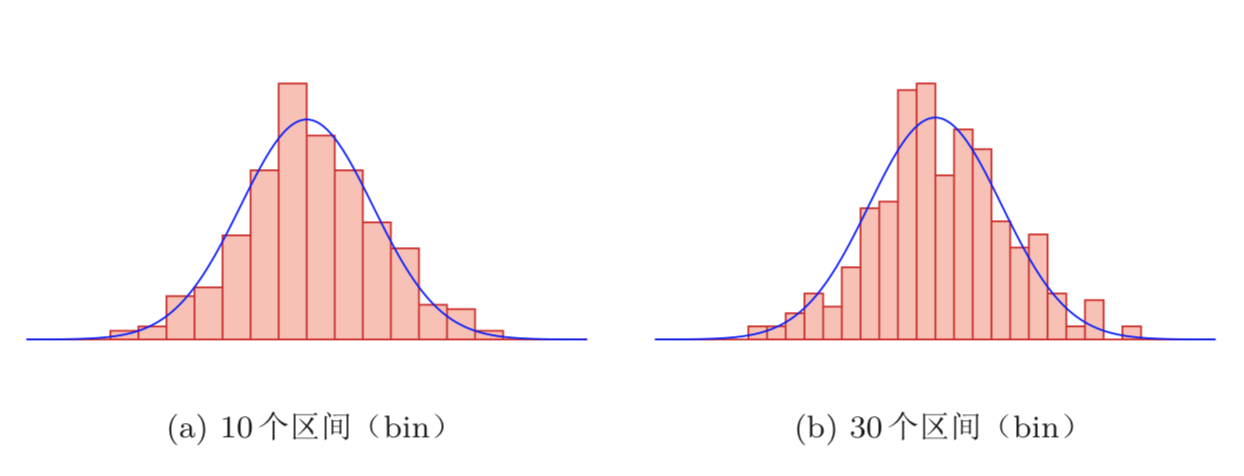##### 核方法

$\phi (\frac{z-x}{h}) = \left\{\begin{matrix} 1 \,\,\,\,\,\, if \, |z_i - x_i|< \frac{h}{2}, 1 \leq i \leq d & \\ 0 \,\,\,\,\,\, else & \end{matrix}\right.$

$K = \sum_{n=1}^{K} \phi (\frac {x^n - x}{h})$

$p(x) = \frac{K}{Nh^d} =\frac{1}{Nh^d} \sum_{n=1}^{K} \phi (\frac {x^n - x}{h})$

$\phi (\frac {z-x}{h}) = \frac {1}{ (2\pi)^{\frac{1}{2}} h} exp(- \frac{||z-x||^2}{2h^2})$

$p (x) = \frac{1}{N} \sum_{n=1}^{N} \frac {1}{ (2\pi)^{\frac{1}{2}} h} exp(- \frac{||z-x||^2}{2h^2})$

##### K近邻方法

K近邻方法也经常用于分类问题，称为K近邻分类器。 当K=1时为最近邻分类器。

## 总结【技术服务】，详情点击查看：https://mp.weixin.qq.com/s/PtX9ukKRBmazAWARprGIAg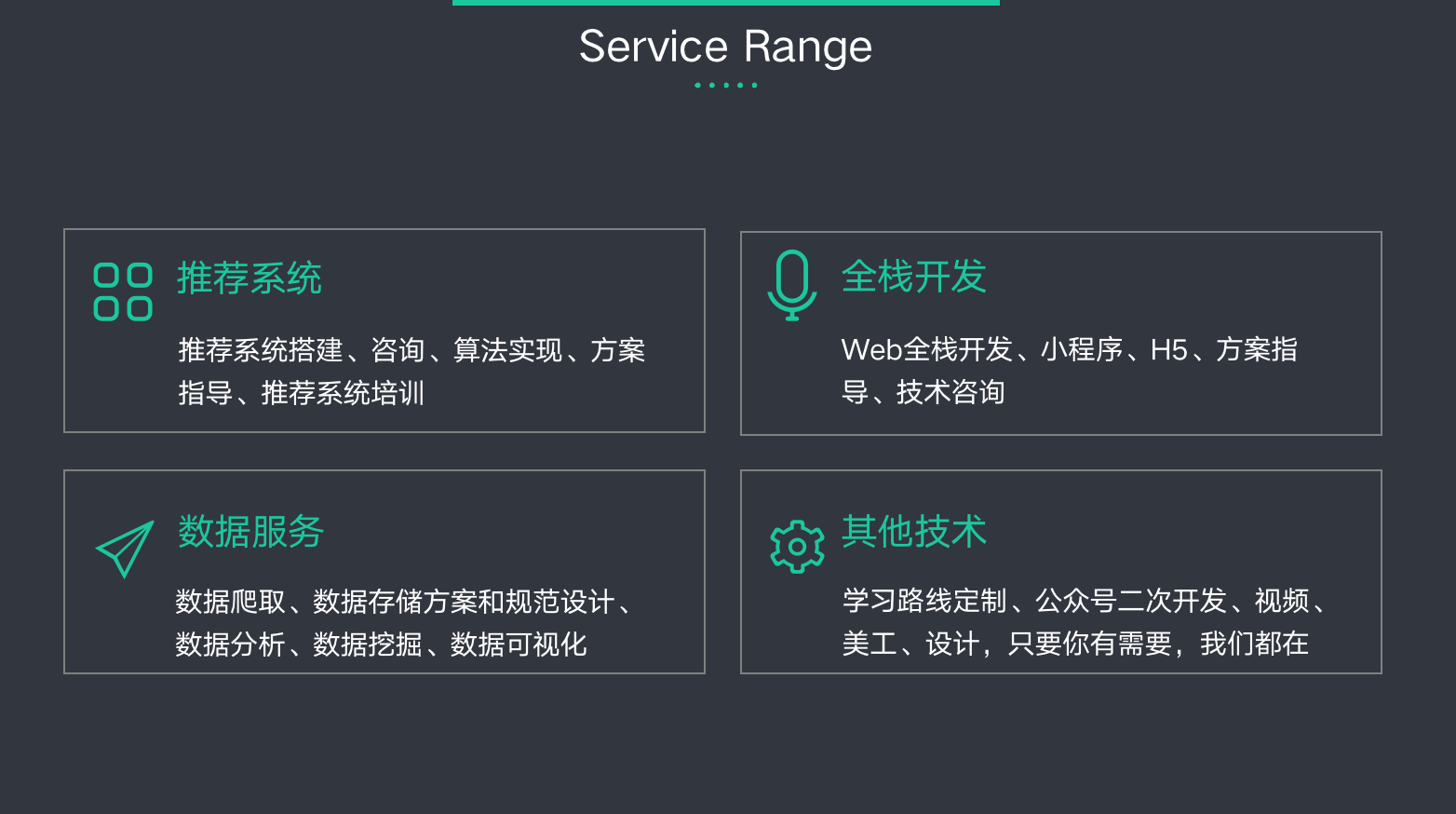©️2019 CSDN 皮肤主题: 大白 设计师: CSDN官方博客